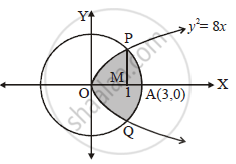# Find the area of the region common to the circle x2 + y2 =9 and the parabola y2 =8x - Mathematics and Statistics

Find the area of the region common to the circle x2 + y2 =9 and the parabola y2 =8x

#### Solution

x^2+y^2=9   and y^2=8x

x^2+8x=9

x^2+8x-9=0

therefore (x+9)(x-1)=0

therefore x=1 or x=-9

therefore y=+-2sqrt2

∴ The points of intersections are

P(1,2sqrt2) and Q(1,-2sqrt2)

y^2=8x

therefore y=sqrt8sqrtx=2sqrt2 x^(1/2)-> f_1(x)

and x^2+y^2=9 therefore y^2=9-x^2

therefore y=sqrt(9-x^2)-> f_2(x)

Required area,
= Area OPAQO = 2 Area OPAMO
= 2(Area OPMO + Area APMA)=2[int_0^1f_1(x)dx+int_1^3f_2(x)dx]

=2[int_0^12sqrt2 x^(1/2)dx+int_1^3sqrt(9-x^2)dx]

=2[2sqrt2(x^(3/2)/(3/2))_0^1+(x/2sqrt(9-x^2)+9/2sin^-1(x/3)_1^3)]

=2[(4sqrt2)/3+(3/2(0)+9/2sin^-1 (1)-1/2sqrt8-9/2sin^-1 (1/3))]

=2[(4sqrt2)/3+9/2.x/2-sqrt2-9/2sin^-1 (1/3)]

=2[(sqrt2/3+(9pi)/4-9/2 sin^-1 (1/3)) sq. units

Concept: Area of the Region Bounded by a Curve and a Line
Is there an error in this question or solution?